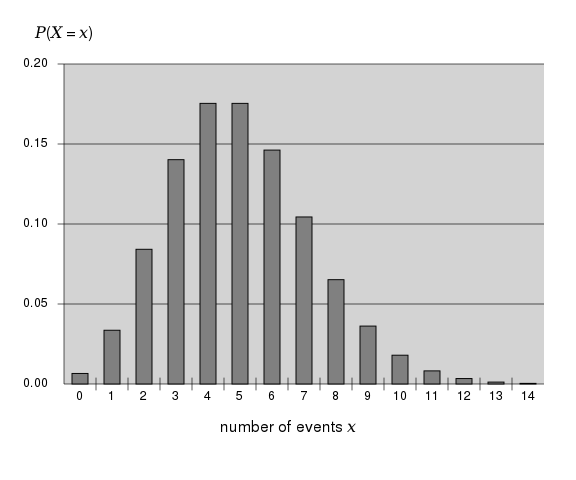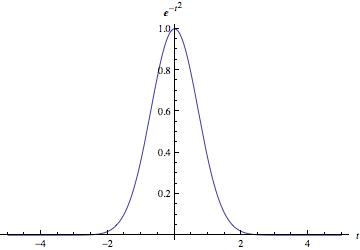# Probability Distribution

## What is Probability Distribution?

Probability distribution is the sum of the probabilities of the events occurring. For a set to qualify as a probability distribution, every value must be mutually exclusive, meaning the events cannot contain any common results. For example, the set (1,2,3,4,5) qualifies as a distribution, while (1,2,3,3,3,5) does not. In the first set, the probability that any event will occur is 4/5, whereas the likelihood that the mutually exclusive event "2" will happen is 1/5. There are two distinct types of probability distributions, continuous and discrete.

### Discrete Probability Distribution

A discrete probability distribution looks at events that occur within a countable sample space. An example of this is throwing dice, where there is a finite and countable set of outcomes. Each possible number on which the die could land is assigned a value, and represented by a set of mutually exclusive probabilities. With one die, the chances of rolling any given number is 1/6.By Alvaro Vidal-Abarca - Own work, CC BY-SA 4.0, https://commons.wikimedia.org/w/index.php?curid=50783781

### Continuous Probability Distribution

A continuous probability distribution looks at events that occur within a sample space that is less defined. Often a continuous variable is one that is achieved by measuring (e.g. height of students in a class, time it takes to get to work). These variables are less static and may not always be represented by whole numbers.By Atakbi1 - Own work, CC BY-SA 3.0, https://commons.wikimedia.org/w/index.php?curid=23068806